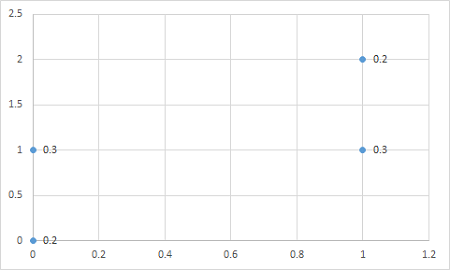# Let X and Y have the joint defined by f(0, 0) = f(1, 2) = 0.2, f(0, 1) = f(1, 1) = 0.3. Depict...

## Question:

Let X and Y have the joint defined by f(0, 0) = f(1, 2) = 0.2, f(0, 1) = f(1, 1) = 0.3. Depict the points and corresponding probabilities on a graph. Give the marginal pmfs in the "margins" Compute mu_x, mu_y, Find the equation of the least squares regression line and draw it on your graph. Does the line make sense to you intuitively?

## Least-Squares Regression Line:

The regression line is calculated such that the difference between the observed values and the regression line is as little as possible and that is why it is known as least squares regression line as it tends to minimize the square of the errors.

## Answer and Explanation:

Become a Study.com member to unlock this answer! Create your accountThe marginal PMF of X = {eq}\sum_{y}f_{X,Y}(x,y) {/eq}

{eq}f_{X}(0)=f(0,0)+f(0,1) = 0.2+0.3 = 0.5\\ f_{X}(1)=f(1,2)+f(1,1) = 0.2+0.3 =...

See full answer below.

#### Learn more about this topic:Simple Linear Regression: Definition, Formula & Examples

from

Chapter 8 / Lesson 2
32K

Simple linear regression is a great way to make observations and interpret data. In this lesson, you will learn to find the regression line of a set of data using a ruler and a graphing calculator.